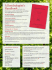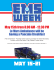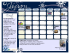# Homework 1 (due March 3)

## Transcription

Homework 1 (due March 3)
```CS 598 JGE
Homework 1
Spring 2011
Homework 1 (due March 3)
Teams of up to three people can turn in joint homework solutions. Complete solutions for each numbered
problem (excluding starred subproblems) should take at most 3 typeset pages. You may use any resource
at your disposal—printed, electronic, or human—but cite/acknowledge your sources, just as though you
were writing a research paper. ∗ Stars indicate problems that I don’t know how to solve; these problems
may or may not actually be open (or interesting).
1. In class we described an extension of Sleator and Tarjan’s link-cut trees that support path operations
via lazy propagation. Specifically, the data structure maintains a value at each node in a dynamic
forest, and supports the following operations in O(log n) amortized time, in addition to the core
• GETVALUE(v): Return the value associated with v.
• SETVALUE(v, x): Change the value associated with v to x.
• MINPATH(v): Return the ancestor of v with minimum value.
• ADDPATH(v, ∆): Add ∆ to the value of every ancestor of v.
Extend link-cut trees further, to support the following subtree operations:
• MINSUBTREE(v): Return the descendant of v with minimum value.
• ADDSUBTREE(v, ∆): Add ∆ to the value of every descendant of v.
The algorithms for LINK, CUT, FINDROOT, GETVALUE, SETVALUE, MINPATH, and ADDPATH should
require only minor modifications; all operations should run in O(log n) amortized time. You may
assume that every node in the represented forest has degree at most 3. (This assumption is not
actually necessary, but it dramatically simplifies the solution.)
1
CS 598 JGE
Homework 1
Spring 2011
2. In the first week of class, we saw a method to transform a data structure that supports queries and
insertions into a data structure that supports queries, insertions, and deletions, when the binary
¯. The basic idea is to maintain both a
operation used to compose query results has an inverse main data structure D and an auxiliary anti-data structure D. To delete an item from the modified
data structure, we actually insert it into D; then, if the size of D exceeds half the size of D, we
rebuild the entire data structure from scratch, discarding the items in D.
However, as one student observed in class, this modified structure has issues with repeated
insertions and deletions of the same item. After inserting and deleting an item, we can’t insert that
item again until after the next global rebuild! The standard dodge is to assume either that repeated
insertions just never happen, or that the underlying data structure actually stores a multiset and
therefore directly supports multiple insertions.
But suppose the original data structure really maintains a set? Specifically, let’s assume the
data structure maintains a set A and supports the following operations:
• INSERT(a) — Insert item a into the data set A. If a ∈ A before the operation, the operation
has no effect. This operation takes I(n) time. Assume that I(n) = Ω(log n).
• MEMBER?(a) — Return TRUE if and only if a ∈ A. This operation takes less than I(n) time.
• QUERY(x) — Answer the decomposable query Q(x, A), in Q(n) time.
Let P(n) denote the preprocessing time to build a new data structure containing n items.
To add support for deletions, we can maintain an exponential sequence D0 , D1 , D2 , D3 , . . . of
alternating data and anti-data structures, where each structure Di stores a subset of at most half
the data in the preceding structure Di−1 . Thus, the actual data set stored in the exponential
structure is
A := D0 \ (D1 \ (D2 \ (D3 \ · · · ))) = (D0 \ D1 ) ∪ (D2 \ D3 ) ∪ · · · ,
and therefore a query can be answered using the alternating ‘sum’
¯ Q(x, D1 ) ¯ Q(x, D2 ) ¯ Q(x, D3 ) ¯ ···
Q(x, A) = Q(x, D0 ) ¯ Q(x, D1 ) Q(x, D2 ) ¯ Q(x, D3 ) · · ·
= Q(x, D0 ) (a) What is the query time for this alternating exponential structure?
(b) Describe INSERT and DELETE algorithms for this alternating exponential structure that run
in O(P(n)/n + I(n)) amortized time. INSERTing an item that is already in A should have no
effect; similarly, DELETEing an item that is not in A should have no effect. [Hint: The INSERT
and DELETE algorithms should be essentially identical. O(P(n)/n + I(n) log n) amortized time
is relatively straightforward, and even O(P(n)/n + I(n) log log n) is not hard, but how to get
rid of that last log log n factor?]
(c) Sketch the modifications necessary to make INSERT and DELETE run in O(P(n)/n + I(n))
worst-case time.
2
CS 598 JGE
Homework 1
Spring 2011
3. After the Great Academic Meltdown of 2020, you get a job as a cook’s assistant at Jumpin’ Jack’s
Flapjack Stack Shack, which sells arbitrarily-large stacks of pancakes for just four bits (50 cents)
each. Jumpin’ Jack insists that any stack of pancakes given to one of his customers must be sorted,
with smaller pancakes on top of larger pancakes. Also, whenever a pancake goes to a customer, its
top side should not be burned.
The cook provides you with a unsorted stack of n perfectly round pancakes, of n different sizes,
possibly burned on one or both sides. Your task is to throw out the pancakes that are burned on
both sides (and only those) and sort the remaining pancakes so that their burned sides (if any)
face down. Your only tool is a spatula. You can insert the spatula under any pancake and then
either flip or discard the stack of pancakes above the spatula.
More concretely, let us represent a stack of pancakes by a sequence of distinct integers between
1 and n, representing the sizes of the pancakes, with each number marked to indicate the burned
side(s) of the corresponding pancake. For example, 1 4 3 2 represents a stack of four pancakes: a
one-inch pancake burned on the bottom; a four-inch pancake burned on the top; an unburned
three-inch pancake, and a two-inch pancake burned on both sides. We store this sequence in a
data structure that supports the following operations:
• POSITION(x): Return the position of integer x in the current sequence, or 0 if x is not in the
sequence.
• VALUE(k): Return the kth integer in the current sequence, or 0 if the sequence has no kth
element. VALUE is essentially the inverse of POSITION.
• TOPBURNED(k): Return TRUE if and only if the top side of the kth pancake in the current
sequence is burned.
• FLIP(k): Reverse the order and the burn marks of the first k elements of the sequence.
(a) Describe an algorithm to filter and sort any stack of n burned pancakes using O(n) of the
operations listed above. Try to make the big-Oh constant small.
1432
FLIP(4)
/ 2341
/ 341
FLIP(2)
/ 431
FLIP(3)
/ 134
(b) Describe a data structure that supports each of the operations listed above in O(log n)
amortized time. Together with part (a), such a data structure gives us an algorithm to filter
and sort any stack of n burned pancakes in O(n log n) time.
? (c)
Æ
Prove or disprove: Sorting unburned pancakes requires at least Ω(log n) amortized time
per operation. More concretely: Any data structure that stores a permutation of the set
{1, 2, . . . , n} and supports the operations POSITION, VALUE, and FLIP requires at least Ω(log n)
amortized time per operation in the cell-probe model. [We’ll talk about cell-probe lower
bounds later in the semester. Meanwhile, the following paper may be relevant: Erik Demaine
and Mihai Pˇ
atra¸scu, Lower Bounds for Dynamic Connectivity, STOC 2004.]
(d) Describe an integer-RAM algorithm that computes, in o(n log n) time, a sequence of O(n)
FLIP operations that sorts a given stack of n unburned pancakes. Alternatively, prove that no
such algorithm exists. [Hint: There are sorting algorithms that run in o(n log n) time on the
integer RAM; that’s not the hard part.]
3
CS 598 JGE
Æ
Homework 1
Spring 2011
4. Link-cut trees (like most other dynamic tree data structures) are built on top of splay trees, but
their analysis does not reflect the rich set of results known for splay trees. Can we prove stronger
results about the efficiency of link-cut trees, or any other dynamic tree data structure, similar to
the static optimality theorem, working set theorem, sequential access theorem, dynamic finger
theorem, and the like for splay trees? Here are some concrete examples:
Suppose we perform an depth-first search of some rooted tree T , calling EXPOSE(v) each time
we visit any node v. For link-cut trees, the analysis in class implies a total running time of at most
O(n log n). However, I believe the sequential access theorem implies that if T is a path, the actual
running time is only O(n). It the total running time always O(n), or does it depend on the tree T ?
Fix a tree T with n nodes and a sequence X of m EXPOSE operations. For each node v, let m v
denote the number of times that EXPOSE(v) appears in X . For
P link-cut trees, the analysis in class
implies a total running time of O(m log n), but is it really O( v m v log(m/m v )), or something else?
Does the actual running time also depend on T , or the given permutation of X ?
Are there interesting lower bounds for dynamic trees (or perhaps I should say self-adjusting
representations of static trees), like Wilber’s lower bounds and their geometric descendants for
These questions are probably very hard, so any partial results are potentially interesting.
4
```

### PANCAKE DAY!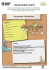### LAFD #71 Pancake Breakfast Flyer 2015.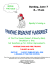### What you`ll need. How to put it all together.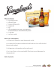### Kruse and Muer on Wilshire Rochester Chop House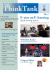### Let’s Make Pancakes Teacher Edition Written by Emma Rossi### Heck Yeah, Banana Pancakes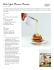### A Dendrologist`s Handbook